Courses

# Helmholtz Free Energy Or Helmholtz Function Or Work Function - Thermochemistry Chemistry Notes | EduRev

## IIT JAM : Helmholtz Free Energy Or Helmholtz Function Or Work Function - Thermochemistry Chemistry Notes | EduRev

The document Helmholtz Free Energy Or Helmholtz Function Or Work Function - Thermochemistry Chemistry Notes | EduRev is a part of the IIT JAM Course Physical Chemistry.
All you need of IIT JAM at this link: IIT JAM

Helmholtz free energy or Helmholtz function or work function.

The work function (A) is defined as

A = U – TS

Let an isothermal change at temperature T from the initial state indicated by subscript (1) to final state subscript (Z) so that
A1 = U– TS1
&              A=  – TS2
A2 – A1 = (U2 – U1) – T(S– S1)
ΔA = ΔU – TΔS
or             dA = dU – TdS

dA = dU – TdS                                                         [∴ TdS = dq]
dA = dU – dq

From first law dq = dU – ω or  dU – dq = ω i.e.
dA = ω

Free energy function Gibb’s free energy function.
The free energy funct ion G is given by G = H – TS
Let us consider the change of system from (1) to (2) at constant temperature, we have

G2 – G1 = (H2 – H1) – T(S– S1)

ΔG = ΔH – TΔS
dG = dH – TdS

Variation of free energy with pressure:

We know that

dG = VdP – SdT

when process is isothermal then

dT = 0

(dG)T = V(dP)T
⇒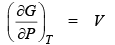When pressure is constant the
dP = 0

(dG) P = –S(dT)P
⇒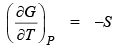Isothermal change in free energy for ideal gas
dG = VdP

Integrating wit h the limit s G1 and G2, P1 and Pwe get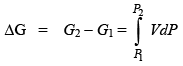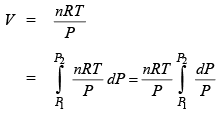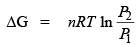we know that P1V1 = P2V2
then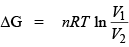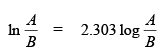Isothermal change in free energy for solid and liquid The volume occupied by solid and liquid is nearly independent of pressure:

dG = VdP
G2 – G= V(P2 – P1)

Problem.  Two mole of an ideal gas are allowed to expand reversibly and isothermally at 300 K from a pressure of 1 atm to a pressure of 0.1 atm.  What is the change is Gibb’s free energy.
Sol.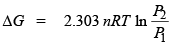= 2.303 x 2 x 8.314 x 300 logΔG = –11488.2 J = –11.4882 KJ

Gibbs Helmholtz equation.

(A) Temperature depends of free energy we know that

G = H – TS

&      dG = VdP – SdT
dG = VdP – SdT

As pressure is constant, therefore

(dG)P = –S(dT)P
∴            dG1 = –S1dT

S1 & S2 are entropy in the init ial and final states.

dG= –S2dT

Subtracting these equation we get
dG2 – dG1 = –(S2 – S1)dT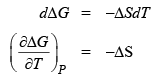&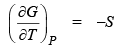We know that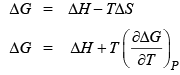…(1)
This is Gibb’s Helmholtz equation.

ΔG = ΔH - T ΔS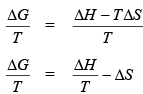Differentiating this equation w.r.t. T at constant P we get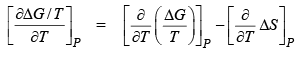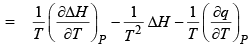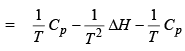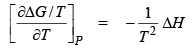... (2)

(B)  Temperature dependence of Helmholtz function:

We know that      A = U – TS
ΔA = ΔU – TΔS
&                         dA = –PdV – SdT

As vo lume is constant, therefore

dA = –SdT dA1 = –S1dT

S1 and S2 are init ial and final entropy. dA2 = –S2dT Subtracting these equation we get
dA2 – dA1 = –(S2 – S1)dT
dΔA = –ΔSdT

⇒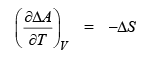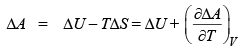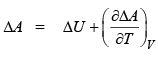This is Gibbs Helmholtz equation.

ΔA = ΔU – TΔS
⇒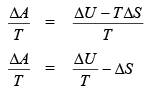Different iat ing this equation w.r.t. T at constant V we get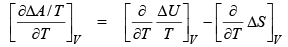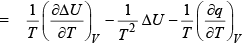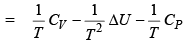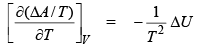…(2)

Problem.  Prove that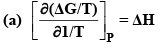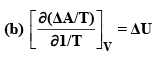Sol. (a) ΔG = ΔH – TΔS dividing by T we get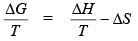differentiation of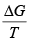by T at constant P, we get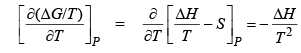or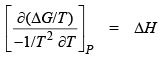Since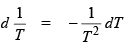therefore, we have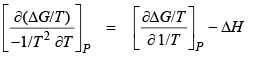i.e.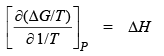Gibbs Helmholtz equation.

(b)  Similarly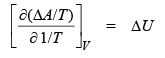Gibbs Helmholtz equation.
Problem.
For the reaction.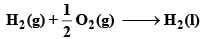The value of enthalpy change and free energy change are –68.32 and –56.69 K cals respectively.

Calculate the value of free energy change at 30°C.
Sol. We know that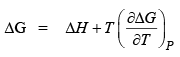T = 25° = 273 + 25 = 298 K
–56.69 = 68.32 298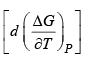⇒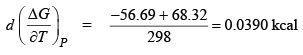then at 30°C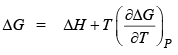= –68.32 + 303 × 0.0390
ΔG = –56.495 kcal.

Offer running on EduRev: Apply code STAYHOME200 to get INR 200 off on our premium plan EduRev Infinity!

## Physical Chemistry

84 videos|106 docs|31 tests

,

,

,

,

,

,

,

,

,

,

,

,

,

,

,

,

,

,

,

,

,

;